# Natural Calculus

The following article is from The Great Soviet Encyclopedia (1979). It might be outdated or ideologically biased.

## Natural Calculus

(or systems of natural deduction), the general name of logical calculi introduced and studied in 1934 by the German logician G. Gentzen (and independently by the Polish logician S. Jaskowski) in order to formalize the process of logical deduction, to reproduce as precisely as possible the structure of ordinary meaningful judgments, and to solve a number of important problems in metamathematics, including a proof of the consistency of the arithmetic of natural numbers.

We may take as the fundamental object of the natural calculus the relation of (formal) deducibility, denoted by the symbol ǀ-, possessing, by definition, the property A ᅡ A (here A is an arbitrary proposition expressed by a formula of the natural calculus) and satisfying a number of “structural” rules of deduction (henceforth, in writing the rules, we place under the horizontal line the deducibility relationship obtained by assuming that we are given the deducibility relationships written above the line; uppercase Latin letters denote arbitrary formulas, and Greek letters denote sequences of formulas). These rules of deduction include the following: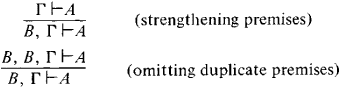and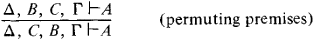The form and number of the structural rules differ in different formulations of the natural calculus. For example, if Δ and Γ are understood to mean not sequences but simply finite (unordered) sets of formulas, we may manage without rules for permuting premises; the usual convention that each element occur in a set only once obviates the need for a rule for omitting duplicate premises and other rules.

Logical rules of deduction that regulate the procedure for introducing and removing (eliminating, excluding) symbols of the logical operations and that describe (like the axioms of “ordinary” logical calculi) the properties of these operations also occur in the natural calculus. The rules of the classical natural propositional calculus are as follows.

(1) Introduction of: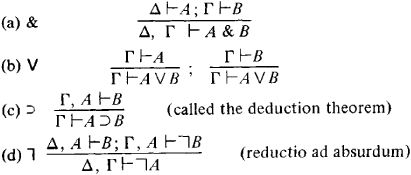(2) Elimination of: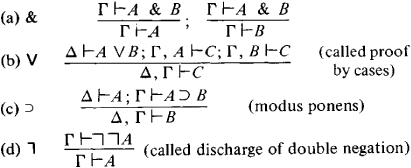We have indicated within the parentheses the interpretation of several of the rules in terms of traditional logic; the interpretation of the other rules is the same as for the corresponding axioms of the ordinary propositional calculus whose reformulations they are.

If we add the corresponding rules of introduction and elimination for quantifiers to this list, then we obtain the natural predicate calculus. The replacement of the 1-elimination rule by the weak Γ-elimination rule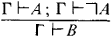(“a contradiction implies any proposition”) leads to the intuitionist (constructive) natural propositional calculus and, with appropriate changes in the quantifier rules, to the intuitionist natural predicate calculus.

A proof in the natural calculus is, as usual, an inference from an empty set of premises. The rule of ⊃-introduction becomes the means of obtaining the “logical rules” expressed by formulas that can be proved without introducing arbitrary hypotheses (premises) in formulations of the natural calculus similar to the formulation presented here in which there are no axioms (other than perhaps, A ᅡ A). The flexibility of the apparatus of the natural calculus, the similarity of the apparatus to ordinary forms of meaningful judgments, and the simplicity of the resulting deductions make it a convenient research tool in mathematical logic. The natural calculus is useful whenever other systems of logic are used and can be the source of derived (additional) rules of deduction whose use also significantly simplifies the logical apparatus. The natural calculus is also useful for obtaining heuristic (preliminary) arguments subject to further proof, which must in any case precede any formal proof (as the source of provable or refutable hypotheses).

### REFERENCES

Kleene, S. C. Vvedenie ν metamatematiku, secs. 20 and 23. Moscow, 1957. (Translated from English.)
Gentzen, G. “Issledovaniia logicheskikh vyvodov.” In Matematicheskaia teoriia logicheskogo vyvoda. Moscow, 1967. (Translated from German.)
Curry, H. B. Osnovaniia matematicheskoi logiki. Moscow, 1969. (Translated from English.)

IU. A. GASTEV